Technical Test SSC JE: Electrical Engineering (EE)- 13

# Technical Test SSC JE: Electrical Engineering (EE)- 13

Test Description

## 100 Questions MCQ Test Mock Test Series for SSC JE Electrical Engineering | Technical Test SSC JE: Electrical Engineering (EE)- 13

Technical Test SSC JE: Electrical Engineering (EE)- 13 for Electrical Engineering (EE) 2022 is part of Mock Test Series for SSC JE Electrical Engineering preparation. The Technical Test SSC JE: Electrical Engineering (EE)- 13 questions and answers have been prepared according to the Electrical Engineering (EE) exam syllabus.The Technical Test SSC JE: Electrical Engineering (EE)- 13 MCQs are made for Electrical Engineering (EE) 2022 Exam. Find important definitions, questions, notes, meanings, examples, exercises, MCQs and online tests for Technical Test SSC JE: Electrical Engineering (EE)- 13 below.
Solutions of Technical Test SSC JE: Electrical Engineering (EE)- 13 questions in English are available as part of our Mock Test Series for SSC JE Electrical Engineering for Electrical Engineering (EE) & Technical Test SSC JE: Electrical Engineering (EE)- 13 solutions in Hindi for Mock Test Series for SSC JE Electrical Engineering course. Download more important topics, notes, lectures and mock test series for Electrical Engineering (EE) Exam by signing up for free. Attempt Technical Test SSC JE: Electrical Engineering (EE)- 13 | 100 questions in 100 minutes | Mock test for Electrical Engineering (EE) preparation | Free important questions MCQ to study Mock Test Series for SSC JE Electrical Engineering for Electrical Engineering (EE) Exam | Download free PDF with solutions
 1 Crore+ students have signed up on EduRev. Have you?
Technical Test SSC JE: Electrical Engineering (EE)- 13 - Question 1

### A monopole consists of

Technical Test SSC JE: Electrical Engineering (EE)- 13 - Question 2

### The dependent current source shown in figure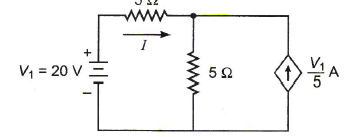Technical Test SSC JE: Electrical Engineering (EE)- 13 - Question 3

### Consider the circuit as shown below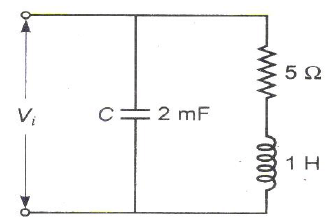The Q factor of the inductor is equal to

Technical Test SSC JE: Electrical Engineering (EE)- 13 - Question 4

If a sinusoidal voltage source is connected to a power transformer, its no load current would be

Technical Test SSC JE: Electrical Engineering (EE)- 13 - Question 5

Short-circuit test is performed on a transformer with a certain impressed voltage at rated frequency. If the short-circuit test is now performed with the same magnitude of impressed voltage but at a frequency higher than the rated frequency, then

Technical Test SSC JE: Electrical Engineering (EE)- 13 - Question 6

If a 230 V DC series motor is connected to a 230 V AC supply, then the motor will

Technical Test SSC JE: Electrical Engineering (EE)- 13 - Question 7

A pair of similar DC shunt generators operate in parallel and supply a common load. It is
required to switch-off machine 1 and allow machine 2 to supply the entire load. The
following operations are to be used to achieve this
1. Switch-off the main switch of machine 1.
2. Reduce the field current of machine 1.
3. Increase the field current of machine 2.
4. Ensure that machine 1 just floats.
The correct sequence of these operations is

Technical Test SSC JE: Electrical Engineering (EE)- 13 - Question 8

Match List-I with List-II and select the correct answer using the codes given below the lists.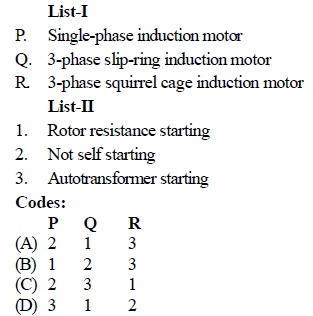Technical Test SSC JE: Electrical Engineering (EE)- 13 - Question 9

As compared to DOL starting, a cage induction motor with star-delta starting shall have

Technical Test SSC JE: Electrical Engineering (EE)- 13 - Question 10

The speed of a 3-phase induction motor operating in its stable region

Technical Test SSC JE: Electrical Engineering (EE)- 13 - Question 11

Match List-I (power factor) with List-II (armature reaction of an alternator) and select
the correct answer using the codes given below the lists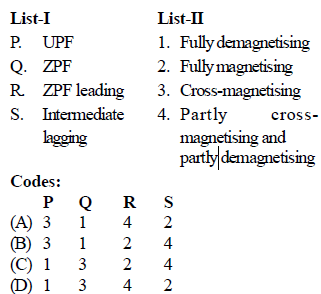Technical Test SSC JE: Electrical Engineering (EE)- 13 - Question 12

While a 2-pole AC servomotor is in operation, if the voltage across the control field winding becomes zero, then the motor has a tendency to run as a single-phase induction motor. To prevent this

Technical Test SSC JE: Electrical Engineering (EE)- 13 - Question 13

Which of the following single-phase motor has highest starting torque

Technical Test SSC JE: Electrical Engineering (EE)- 13 - Question 14

If an AC servomotor has one of its winding excited by AC, then voltage measured at the other winding with rotor running will be

Technical Test SSC JE: Electrical Engineering (EE)- 13 - Question 15

On which one of the following cycles does a modern steam plant work

Technical Test SSC JE: Electrical Engineering (EE)- 13 - Question 16

The most appropriate operating speeds in rpm of generator used in thermal, nuclear and hydropower plants would be

Technical Test SSC JE: Electrical Engineering (EE)- 13 - Question 17

In the network as shown in figure the marked parameters are per unit impedances. The busadmittance matrix of the network is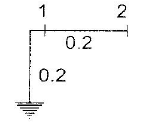Technical Test SSC JE: Electrical Engineering (EE)- 13 - Question 18

The Buchholz relay protects a transformer from

Technical Test SSC JE: Electrical Engineering (EE)- 13 - Question 19

If the fault current is 3000 A for a relay with a plug setting of 50% and CT ratio of 1000 : 1,the plug setting multiplier would be

Technical Test SSC JE: Electrical Engineering (EE)- 13 - Question 20

Maloperation of differential protection of transformers due to magnetizing in rush current is prevented by

Technical Test SSC JE: Electrical Engineering (EE)- 13 - Question 21

In twBo wattmeter method of measuring 3-phase power, power factor is 0.5, then one of the wattmeter will read

Technical Test SSC JE: Electrical Engineering (EE)- 13 - Question 22

Schering bridge is used to

Technical Test SSC JE: Electrical Engineering (EE)- 13 - Question 23

Following figures show four bridges. Which figure is Owen's bridge

Technical Test SSC JE: Electrical Engineering (EE)- 13 - Question 24

The power in a resistor R is estimated measuring the voltage and current using voltmeter-ammeter method. Two different arrangements can be used as shown in circuit I and II. Less erroneous results are obtained adapting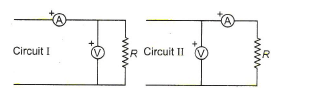Technical Test SSC JE: Electrical Engineering (EE)- 13 - Question 25

A CRO uses

Technical Test SSC JE: Electrical Engineering (EE)- 13 - Question 26

The latching current of SCR is 18 mA. Its holding current will be

Technical Test SSC JE: Electrical Engineering (EE)- 13 - Question 27

The maximum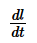in a SCR is

Technical Test SSC JE: Electrical Engineering (EE)- 13 - Question 28

In the figure below the, current of 1A flows through the resistance of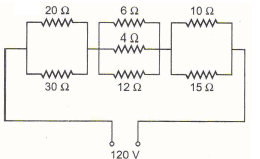Technical Test SSC JE: Electrical Engineering (EE)- 13 - Question 29

Find the value of Vx in the given figure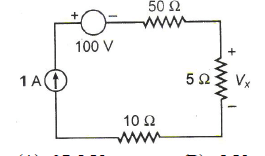Technical Test SSC JE: Electrical Engineering (EE)- 13 - Question 30

Which of the following is correct regarding autotransformer

Technical Test SSC JE: Electrical Engineering (EE)- 13 - Question 31

In a three-phase D/Y transformer shown in the figure, the phase displacement of secondary line voltage with corresponding primary line voltages will be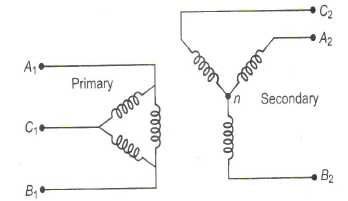Technical Test SSC JE: Electrical Engineering (EE)- 13 - Question 32

If the speed of a DC motor increases with load torque, then it is a

Technical Test SSC JE: Electrical Engineering (EE)- 13 - Question 33

A 3-phase slip-ring induction motor is wound for 4-poles on stator and 6-poles on rotor.When 3-phase balanced voltage source at 50 Hz is applied to the motor, it will run at

Technical Test SSC JE: Electrical Engineering (EE)- 13 - Question 34

The phasor diagram of a synchronous machine connected to an infinite bus is shown in the figure. The machine is acting as a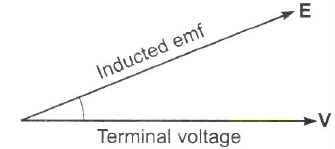Technical Test SSC JE: Electrical Engineering (EE)- 13 - Question 35

Match List-I with List-II and select the correct answer using the codes given below the lists.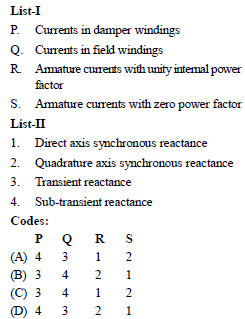Technical Test SSC JE: Electrical Engineering (EE)- 13 - Question 36

Which of the following capacitors start splitphase induction motors will have the largest value of capacitance

Technical Test SSC JE: Electrical Engineering (EE)- 13 - Question 37

An AC servomotor exhibits

Technical Test SSC JE: Electrical Engineering (EE)- 13 - Question 38

In hydro power stations, what is an enlarged body of water just above the in take and used as a regulating reservoir

Technical Test SSC JE: Electrical Engineering (EE)- 13 - Question 39

Resistance switching is normally resorted in case of

Technical Test SSC JE: Electrical Engineering (EE)- 13 - Question 40

A Mho relay is a

Technical Test SSC JE: Electrical Engineering (EE)- 13 - Question 41

Match List-I (Relays) with List-II (Protection) and select the correct answer using the codes
given below the lists: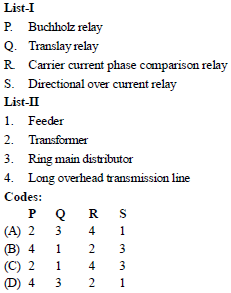Technical Test SSC JE: Electrical Engineering (EE)- 13 - Question 42

The limiting errors of measurement of power consumed by and the voltage error resistanceare + 2% and + 3.5% respectively. The limiting error of measurement of resistance will be

Technical Test SSC JE: Electrical Engineering (EE)- 13 - Question 43

In a gravity controlled instrument, the deflection angle is proportional to

Technical Test SSC JE: Electrical Engineering (EE)- 13 - Question 44

The problem of electro-static coupling in a transformer is acute at

Technical Test SSC JE: Electrical Engineering (EE)- 13 - Question 45

For power measurement of three-phase circuit by two wattmeter method, when the value of power factor is less than 0.5 lagging

Technical Test SSC JE: Electrical Engineering (EE)- 13 - Question 46

Which bridge is used to determine frequency

Technical Test SSC JE: Electrical Engineering (EE)- 13 - Question 47

Match List-I with List-II and select the correct answer using the codes given below the lists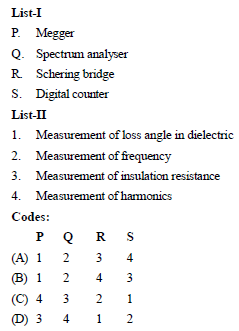Technical Test SSC JE: Electrical Engineering (EE)- 13 - Question 48

In the Owen's bridge shown in below figure,Z1 = 200 Ð 60º, Z2 = 400 Ð – 90º, Z3 = 300Ð 0º,Z4 = 400 Ð 30º. Then, choose the correct statement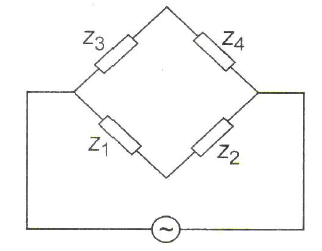Technical Test SSC JE: Electrical Engineering (EE)- 13 - Question 49

Match List-I (Speed/torque regime in a DC motor) with List-II (The control methods
suitable for the same) and select the correct answer using the codes given below the lists.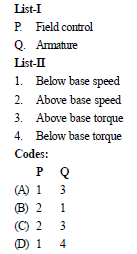Technical Test SSC JE: Electrical Engineering (EE)- 13 - Question 50

In the circuit shown below, diodes have cut-in voltage of 0.6 V. The diode in On state is/are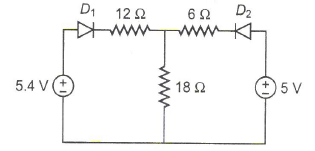Technical Test SSC JE: Electrical Engineering (EE)- 13 - Question 51

In the circuit of the figure, the value of the voltage source E is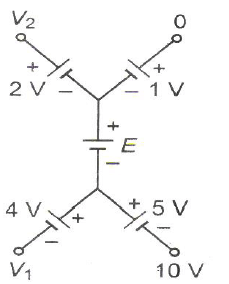Technical Test SSC JE: Electrical Engineering (EE)- 13 - Question 52

In a nuclear power station using boiling water reactor, water is used as

Technical Test SSC JE: Electrical Engineering (EE)- 13 - Question 53

In a DC transmission line

Technical Test SSC JE: Electrical Engineering (EE)- 13 - Question 54

A heater connected to 230 V main supply draws a current of 7.5 A, then what is the
resistance of heating element

Technical Test SSC JE: Electrical Engineering (EE)- 13 - Question 55

Find the equivalent resistance between the nodes P and Q in both of the different cases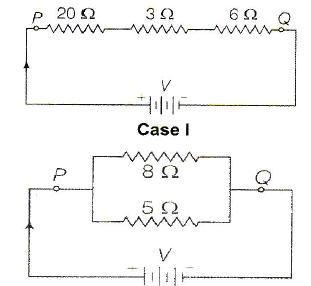Technical Test SSC JE: Electrical Engineering (EE)- 13 - Question 56

The resistance of eureka wire is 2.5 W. What is the value of specific resistance of wire of 14m length and diameter of 0.14 cm

Technical Test SSC JE: Electrical Engineering (EE)- 13 - Question 57

In the figure, a carbon resistor has band of different colours on its body. The resistance of the following body is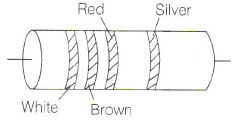Technical Test SSC JE: Electrical Engineering (EE)- 13 - Question 58

A current of 16A is distributed in a circuit having two branches when connected on 220 V supply. If the resistance of one branch is 20W,then find the resistance of other branch and power taken by both the branches.

Technical Test SSC JE: Electrical Engineering (EE)- 13 - Question 59

Determine the electrical energy of a circuit, if the current flowing from the source is 5A for duration of 2s and the resistance of the circuit is 5W

Technical Test SSC JE: Electrical Engineering (EE)- 13 - Question 60

In which of the following, it is not desired to attain the condition of maximum power transfer

Technical Test SSC JE: Electrical Engineering (EE)- 13 - Question 61

For which type of the following Thevenin's and Norton's equivalents cannot be developed

Technical Test SSC JE: Electrical Engineering (EE)- 13 - Question 62

Two two-port networks are connected in cascade. The combination is to represent as a single two-port networks. The parameters of the network are obtained by multiplying the individual

Technical Test SSC JE: Electrical Engineering (EE)- 13 - Question 63

Consider the following statements
1. Interpole windings are connected in series with armature winding
2. Polarity of interpole must be the same as that of the main pole in advance
3. Distortion of the main field under the pole shoes is not affected by the use of interpoles.

Of these statements regarding inter pole used in DC motors, the correct statement(s) is/are

Technical Test SSC JE: Electrical Engineering (EE)- 13 - Question 64

'Crawling' in an induction motor is due to

Technical Test SSC JE: Electrical Engineering (EE)- 13 - Question 65

Match List-I (type of single-phase motor) with List-II (type of appliance) and select the correct answer using the codes given below the lists.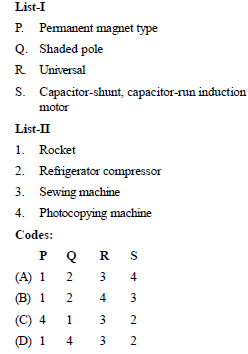Technical Test SSC JE: Electrical Engineering (EE)- 13 - Question 66

A capacitor-start single-phase induction motor is switched-on to supply with its capacitor replaced by an inductor of equivalent reactance value. It will

Technical Test SSC JE: Electrical Engineering (EE)- 13 - Question 67

Match List-I (Classification of head) with List- II (Types of turbine) and select the correct
answer using the codes given below the lists.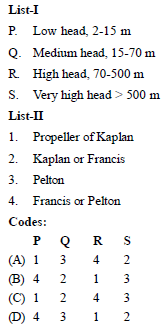Technical Test SSC JE: Electrical Engineering (EE)- 13 - Question 68

In a nuclear power station using boiling water reactor, water is used as

Technical Test SSC JE: Electrical Engineering (EE)- 13 - Question 69

A thermal power plant has installed capacity of 15000 MW. The annual load factor is 60% and capacity factor is 50%. The reverse capacity of the plant will be

Technical Test SSC JE: Electrical Engineering (EE)- 13 - Question 70

If X is the system reactance and R is the resistance, the power transferred is maximum when

Technical Test SSC JE: Electrical Engineering (EE)- 13 - Question 71

Match List-I (Types of relays) with List-II (Types of protection) and select the correct
answer using the codes given below the lists.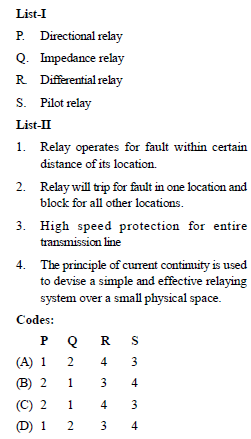Technical Test SSC JE: Electrical Engineering (EE)- 13 - Question 72

The CT supplies current to the current coil of a wattmeter power factor meter, energy meter and an ammeter. These are connected as

Technical Test SSC JE: Electrical Engineering (EE)- 13 - Question 73

Wien’s bridge is useful for measuring

Technical Test SSC JE: Electrical Engineering (EE)- 13 - Question 74

X and Y plates of a CRO are connected to unequal voltages of equal frequency with phase shift of 90°. The Lissajaus figure on the screen will be

Technical Test SSC JE: Electrical Engineering (EE)- 13 - Question 75

R1 and R4 are the opposite arms of a Wheat stone bridge as are R3 and R2. The source voltage is applied across R1 and R3. Under balanced conditions which one of the following is true?

Technical Test SSC JE: Electrical Engineering (EE)- 13 - Question 76

A series circuit has two pure elements with entire network voltage and current equation as

V= 10 sin (l00 t + 80°) VI= 5 cos (l00 t + 50°)

A Then the two elements in the network will be

Technical Test SSC JE: Electrical Engineering (EE)- 13 - Question 77

Consider the following statements in respect of important features of IGBT
1. It has a high impedance gate as that of a MOSFET.
2. It has a low on state voltage as that of a BJT.
3. It can block negative voltages as in the case of GTO.
Which of these statements are correct?

Technical Test SSC JE: Electrical Engineering (EE)- 13 - Question 78

In the figure shown if we connect 2 V with internal resistance 1 Ω at AA’ with positive terminal at A’, the current through R in ampere is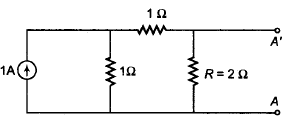Technical Test SSC JE: Electrical Engineering (EE)- 13 - Question 79

Find voltmeter reading of the circuit shown below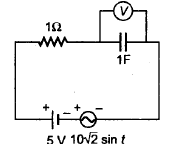Technical Test SSC JE: Electrical Engineering (EE)- 13 - Question 80

The power supplied to the circuit in figure, by the voltage and current sources respectively are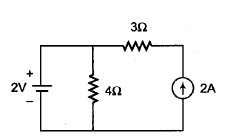Technical Test SSC JE: Electrical Engineering (EE)- 13 - Question 81

If a fixed amount of power is to be transmitted over a certain length with fixed power loss, it can be said that volume of conductor is

Technical Test SSC JE: Electrical Engineering (EE)- 13 - Question 82

Consider the following network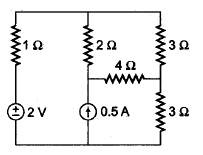The power dissipated across 4 Ω resistor is

Technical Test SSC JE: Electrical Engineering (EE)- 13 - Question 83

The component of excitation current present in Δ-connected secondary of transformer are

Technical Test SSC JE: Electrical Engineering (EE)- 13 - Question 84

In a loaded DC motor, if the bushes are given a shift from the inter polar axis in the direction of relation, then the communication will

Technical Test SSC JE: Electrical Engineering (EE)- 13 - Question 85

The time constant for the given circuit in second is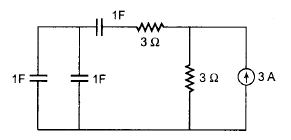Technical Test SSC JE: Electrical Engineering (EE)- 13 - Question 86

In a thyristor, which of the following is correct?

Technical Test SSC JE: Electrical Engineering (EE)- 13 - Question 87

Once SCR starts conducting forward current, its gate losses control over

Technical Test SSC JE: Electrical Engineering (EE)- 13 - Question 88

For parallel operation of transformers, which  condition is essential?
1. Same voltage ratio.
2. Same pu leakage impedance.
3. Same Xe|re ratio.
4. Same polarity.

Technical Test SSC JE: Electrical Engineering (EE)- 13 - Question 89

Match List-I with List-II and select the correct answer using the codes given below the lists.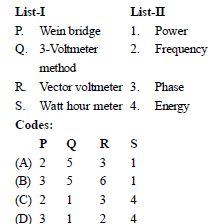Technical Test SSC JE: Electrical Engineering (EE)- 13 - Question 90

A galvanometer of resistance 15 Ω gives full scale deflection for a current of 2 mA. Calculate the shunt resistance needed to convert it to an ammeter of range 0 to 5 A.

Technical Test SSC JE: Electrical Engineering (EE)- 13 - Question 91

A moving coil galvanometer has a resistance of 25 Ω and gives a full scale deflection for a currentof 10 mA. How will you convert it into voltmeter having range 0–100 V?

Technical Test SSC JE: Electrical Engineering (EE)- 13 - Question 92

Two transformers of different kVA ratings working in parallel, share the load in proportionto their ratings when their

Technical Test SSC JE: Electrical Engineering (EE)- 13 - Question 93

In a transformer zero voltage regulation at fullload is

Technical Test SSC JE: Electrical Engineering (EE)- 13 - Question 94

The torque speed characteristic of a repulsion motor resembles which of the following DC motor characteristic

Technical Test SSC JE: Electrical Engineering (EE)- 13 - Question 95

A D/D connected transformer is connected to V/V connected transformer. The ratio of VA rating of V/V connected transformer and D/D connected transformer is

Technical Test SSC JE: Electrical Engineering (EE)- 13 - Question 96

Consider the following statements:

The armature torque in a DC motor is a function of
1. Field flux
2. Armature current
3. Speed
4. Damping
Which of the above statements is/are correct

Technical Test SSC JE: Electrical Engineering (EE)- 13 - Question 97

Insulating materials have the function of

Detailed Solution for Technical Test SSC JE: Electrical Engineering (EE)- 13 - Question 97

The most important function of the insulating material is to insulate voltage-carrying conductors against one another as well as against earth. But, in addition, they must fre- quently perform mechanical functions and must be in a position to withstand certain thermal and chemical stresses.

Technical Test SSC JE: Electrical Engineering (EE)- 13 - Question 98

A 50 Hz, 3-phase synchronous generator has inductance per phase of 15 mH. The capacitance of generator and circuit beaker is 0.002 mF. What is its natural frequency of oscillation

Technical Test SSC JE: Electrical Engineering (EE)- 13 - Question 99

Relative permeability of iron is 4000. What is its magnetic susceptibility

Technical Test SSC JE: Electrical Engineering (EE)- 13 - Question 100

(i) The peak voltage of an AC supply is 300V. What is the rms voltage
(ii) The rms value of current in an AC circuit is 10A. What is the peak current

## Mock Test Series for SSC JE Electrical Engineering

2 videos|1 docs|55 tests
 Use Code STAYHOME200 and get INR 200 additional OFF Use Coupon Code
Information about Technical Test SSC JE: Electrical Engineering (EE)- 13 Page
In this test you can find the Exam questions for Technical Test SSC JE: Electrical Engineering (EE)- 13 solved & explained in the simplest way possible. Besides giving Questions and answers for Technical Test SSC JE: Electrical Engineering (EE)- 13, EduRev gives you an ample number of Online tests for practice

## Mock Test Series for SSC JE Electrical Engineering

2 videos|1 docs|55 tests